Back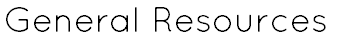Websites Cool Math Games Math Playground Fun Brain ABCYa Common Core Sheets Worksheet Works Math-Aids Purple Math Bite Size Khan Academy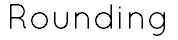MAFS.3.NBT.1.1: Use place value understanding to round whole numbers to the nearest 10 or 100. Rounding to the Nearest 10 Rounding Comparing Numbers Place Value Rounding Place Value Order Whole Numbers Estimate Whole Numbers Math Antics - Rounding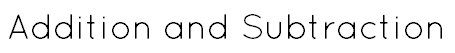MAFS.3.NBT.1.2: Fluently add and subtract within 1000 using strategies and algorithms based on place value, properties of operations, and/or the relationship between addition and subtraction. Associative Property Commutative Property Adding with Regrouping Subtracting with Regrouping Estimate Sums and Differences Add with Regrouping Subtract with Regrouping Relate Addition and Subtraction Properties of Addition Multi-Digit Addition Multidigit Subtraction Borrow Across Zeroes Shortcut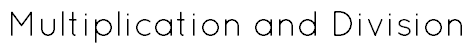MAFS.3.OA.1.1: Interpret products of whole numbers e.g., interpret 5 x 7 as the total number of objects in 5 groups of 7 objects each. MAFS.3.OA.1.2: Interpret whole-number quotients of whole numbers, e.g., interpret 56 ÷ 8 as the number of objects in each share when 56 objects are partitioned equally into 8 shares, or as a number of shares when 56 objects are partitioned into equal shares of 8 objects each. MAFS.3.OA.1.3: Use multiplication and division within 100 to solve word problems in situations involving equal groups, arrays, and measurement quantities, e.g., by using drawings and equations with a symbol for the unknown number to represent the problem. MAFS.3.OA.1.4: Determine the unknown whole number in a multiplication or division problem relating three whole numbers, e.g., 24 = 6 x ♥ MAFS.3.OA.2.5: Apply properties of operations as strategies to multiply and divide. MAFS.3.OA.2.6: Understand division as an unknown-factor problem. MAFS.3.OA.3.7: Fluently multiply and divide within 100, using strategies such as the relationship between multiplication and division (e.g., knowing that 8 x 5 = 40, one knows 40 ÷ 5 = 8) or properties of operations. By the end of Grade 3, know from memory all products of two one-digit numbers. Subtraction Across Zeros Rounding to the Nearest 10 Associative Property of Multiplication Distributive Property of Multiplication Commutative Property of Multiplication Interpret Products of Whole Numbers Interpret Quotients of Whole Numbers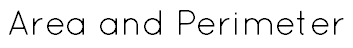MAFS.3.MD.3.5: Recognize are as an attribute of plane figures and understand concepts of area measurement. A square with side length 1 unit, called a "unit square," is said to have "one square unit" of area, and can be used to measure area. A plane figure which can be covered without gaps or overlaps by n unit squares is said to have an area of n square units. MAFS.3.MD.3.6: Measure areas by counting unit squares (square cm, squar m, square in, square ft, and improvised units. MAFS.3.MD.3.7: Relate area to the operations of multiplication and addition. Find the area of a rectangle with whole-number side lengths by tiling it, and show that the area is the same as would be found by multiplying the side lengths. Multiply side lengths to find areas of rectangles with whole-number side lengths in the context of solving real world and mathematical problems, and represent whole-number products as rectangular areas in mathematical reasoning. Use tiling to show in a concrete case that the area of a rectangle with whole-number side lengths a and b+c is the sum of a x b and a x c. Use area models to represent the distributive property in mathematical reasoning. MAFS.G.1.2: Partition shapes into parts with equal areas. Express the area of each part as a unit fraction of the whole. Math Antics - Perimeter Math Antics - Area Math Antics - Distributive Property Study Jams- Perimeter Study Jams- Area of a Parallelogram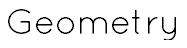MAFS.3.G.1.1: Understand that shapes in different categories (e.g., rhombuses, rectangles, and others) may share attributes (e.g., having four sides), and that the shared attributes can define a larger category (e.g., quadrilaterals). Recongize rhombuses, rectangles, and squares as examples of quadrilaterals, and draw examples of quadrilaterals that do not belong to any of these subcategories. Math Antics - Quadrilaterals Study Jams - Classify Quadrilaterals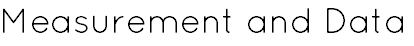MAFS.3.MD.1.1.: Tell and write time to the nearest minute and measure time intervals in minutes. Solve word problems involving addition and subtraction of time intervals in minutes, e.g., by representing the problem on a number line diagram. MAFS.3.MD.2.3: Draw a scaled picture graph and a scaled bar graph to represent a data set with several categories. Solve one- and two-step "how many more" and "how many less" problems using information presented in scaled bar graphs. MAFS.3.MD.2.4: Generate measurement data by measuring lengths using rulers marked with halves and fourths of an inch. Show the data by making a line plot, where the horizontal scale is marked off in appropriate units- whole numbers, halves, or quarters. Study Jams- Telling Time Study Jams- Elapsed Time Study Jams- Units of Measurement Study Jams- Measure Length Study Jams- Add and Subtract Measurements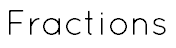MAFS.3.NF.1.1: Understand a fraction 1/b as the quantity formed by 1 part when a whole is partitioned into b equal parts; understand a fraction a/b as the quantity formed by a parts of size 1/b. MAFS.3.NF.1.2: Understand a fraction as a number on the number line; represent fractions on a number line; represent fractions on a number line diagram. Represent a fraction 1/b on a number line diagram by defining the interval from 0 to 1 as the whole and partitioning it into b equal parts. Recognize that each part has size 1/b and that the endpoint of the part based at 0 locates the number 1/b on the number line. Represent a fraction a/b on a number line diagram by marking off a lengths 1/b from 0. Recognize that the resulting interval has size a/b and that its endpoint locates the number a/b on the number line. MAFS.3.NF.1.3: Explain equivalence of fractions in special cases, and compare fractions by reasoning about their size. Understand two fractions as equivalent (equal) if they are the same size, or the same point on a number line. Recognize and generate simple equivalent fractions, e.g., 1/2 = 2/4, 4/6 = 2/3). Explain why the fractions are equivalent, e.g., by using a visual fraction model. Express whole numbers as fractions, and recognize fractions that are equivalent to whole numbers. Examples: Express 3 in the form 3 = 3/1; recognize that 6 = 6/1; locate 4/4 and 1 at the same point of a number line diagram. Compare two fractions with the same numerator or the same denominator by reasoning about their size. Recognize that comparisons are valid only when the two fractions refer to the same whole. Record the results of comparisons with the symbols >, =, or < and justify the conclusions, e.g., by using a visual fraction model. MAFS.G.1.2: Partition shapes into parts with equal areas. Express the area of each part as a unit fraction of the whole. StudyJams - Fractions StudyJams - Simplest Form StudyJams - Equivalent Fractions StudyJams - Finding a Fraction of a Set Introduction to Fractions Math Antics - Fractions are Parts Math Antics - Working with Parts Math Antics - Fractions are Division Math Antics - Types of Fractions Mrs. Munafo - Equivalent Fractions